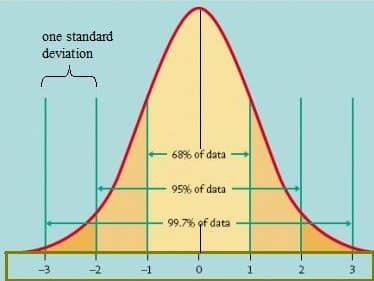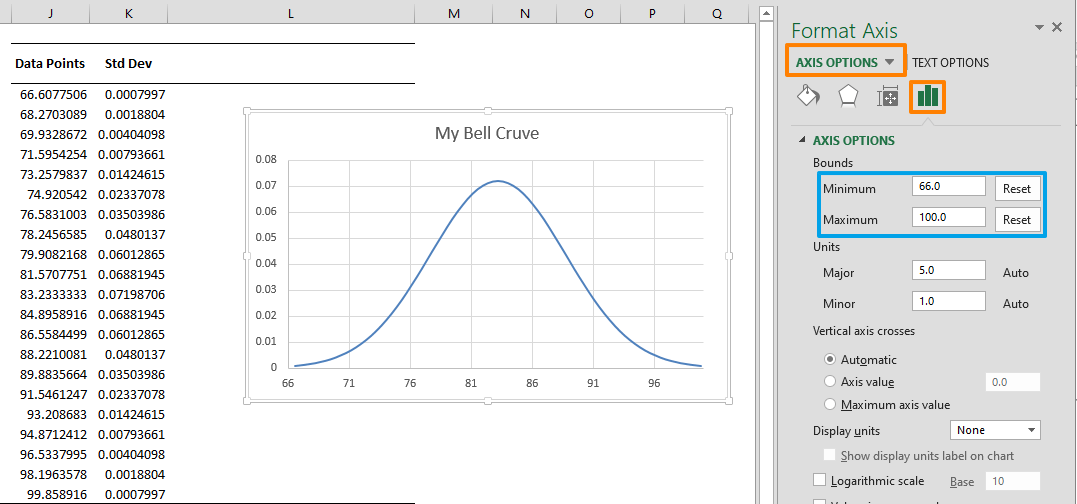A bell curve, also known as normal distribution, is the most common type of distribution for a variable. It is accepted as a distribution that occurs naturally in many situations. In a bell curve, the highest point of the arc defines the mean, which is the highest number of occurrences of an element. The probability of occurrences decrease towards either side of the curve. You can use a bell curve to compare performances by excluding extremes, or define your expectations by the possibility that an outcome will lie within a range to the left or right of the center. In this guide, we are going to show you how to create a bell curve in Excel with a real world use case scenario as an example.

## Basics

All you need is the mean (average) and the standard deviation values of your data set. Both of these metrics can be calculated in Excel using the formulas below.

To calculate mean (average) =AVERAGE(data)

To calculate standard deviation =STDEV.P(data)

In consideration of these two values, normally distributed values follow these rules:

1. The total area under the curve is equal to 1 (100%)
2. The center of the bell curve is the mean of the data point
3. (1-σ) About 68.2% of the area under the curve falls within one standard deviation (Mean ± Standard Deviation)
4. (2-σ) About 95.5% of the area under the curve falls within two standard deviations (Mean ± 2 * Standard Deviation)
5. (3-σ) About 99.7% of the area under the curve falls within three standard deviations (Mean ± 3 * Standard Deviation)Image from University of Virginia

## Creating a bell curve in Excel

Let's take a common example, and say we are analyzing exam results for a class of students. We will be using a bell curve to measure exam results for better comparison.

We begin by calculating the metrics to generate a normal distributed data which will generate our curve. We need to calculate:

• Mean (average) of the values.
• Standard deviation of the values.
• 3-standard deviation limits for before and after mean.
• Interval value for normally distributed data points. This also requires a determination of the interval points. You can select any number, but keep that in mind, more intervals mean more precision.

A quick note before diving into the formulas. We used named ranges instead of cell references to make it easier to read the formulas. You can find more details about named ranges here: Excel Named Ranges

### Metrics

Begin by calculating the mean and standard deviation of the data. You can use the AVEREAGE and STDEV.P functions to calculate the mean and standard deviation values respectively.Next step is calculating the 3 standard deviation values to set the minimum and maximum values for the 99.7% of the data.

The 3 standard deviation values can exceed the actual data set. This is a normal behavior that can occur frequently with smaller data sets.Minimum = 83.23 - 3 * 5.54 = 66.61

Maximum = 83.23 - 3 * 5.54 = 99.86

After setting the minimum and maximum values for our curve, we need to generate the intervals. The interval values will be the base for the normally distributed values. To calculate the intervals, all you need to do is to divide the area between the minimum and maximum values by interval count. In this example, we set this to 20, but you can use a larger number to increase the number of data points.Interval value = (99.85 - 66.60) / 20 = 1.66

Once the interval value is calculated, you can generate the data points. To do this, enter the minimum value in a cell. Then, right below the minimum value, enter the formula to add the interval value to the minimum. Here, we used cell references (like as J4) which helps populate the data points easily up to the maximum value.The next step is to calculate the normally distributed values from the generated data points. You can use Excel's NORM.DIST function to generate these values.

Use the populated data points as the first argument of the function. The mean and standard deviation values are next arguments. Finish the formula with a FALSE Boolean value to use non-cumulative type of this function.### Chart

We're almost done! Select the data points and normal distribution values, then insert an X-Y Scatter chart. Use the Scattered with Smooth Lines version to create a bell curve in Excel.The chart may seem a bit off first. Let's see how you can make it look better.

To change the title of the chart, double-click on the title and update the name.

Next, double-click on the X-axis and define minimum and maximum values from the Axis Options panel to eliminate the white space on both sides. This will give your chart a better bell shape. We set values that are a bit outside our data set. For example, 66 – 100 for values 66.30 – 99.86.You can further enhance your chart by adding the standard deviation values.